Learn from home

The teachersGiven= (2, 1, 3),= (1, −1, 0) and= (1, 2, 3), find the vector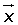= 2u + 3v − w.= (4, 2, 6) + (3, −3, 0) − (1, 2, 3) = (6, −3, 3)

Given vectors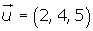and, determine the magnitude of the vector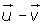.Associative.+ (+) = (+) +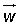Commutative.+=++=+ (−) =### Scalar Multiplication

The product of a number kby a vectoris another vector:

In the same direction asif k is positive.

In opposite direction asif k is negative.

Of magintude.## Properties

Associative.

k · (k' ·) = (k · k') ·Right distributivity.

k · (+) = k ·+ k ·Left distributivity.

(k + k') ·= k ·+ k' ·Multiplicative Identity.

1 ·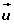=## Example

Given= (6, 2, 0), determineso that 3=.Did you like the article?(1 votes, average: 5.00 out of 5)Loading...

Emma

I am passionate about travelling and currently live and work in Paris. I like to spend my time reading, gardening, running, learning languages and exploring new places.

Did you like
this resource?

Bravo!